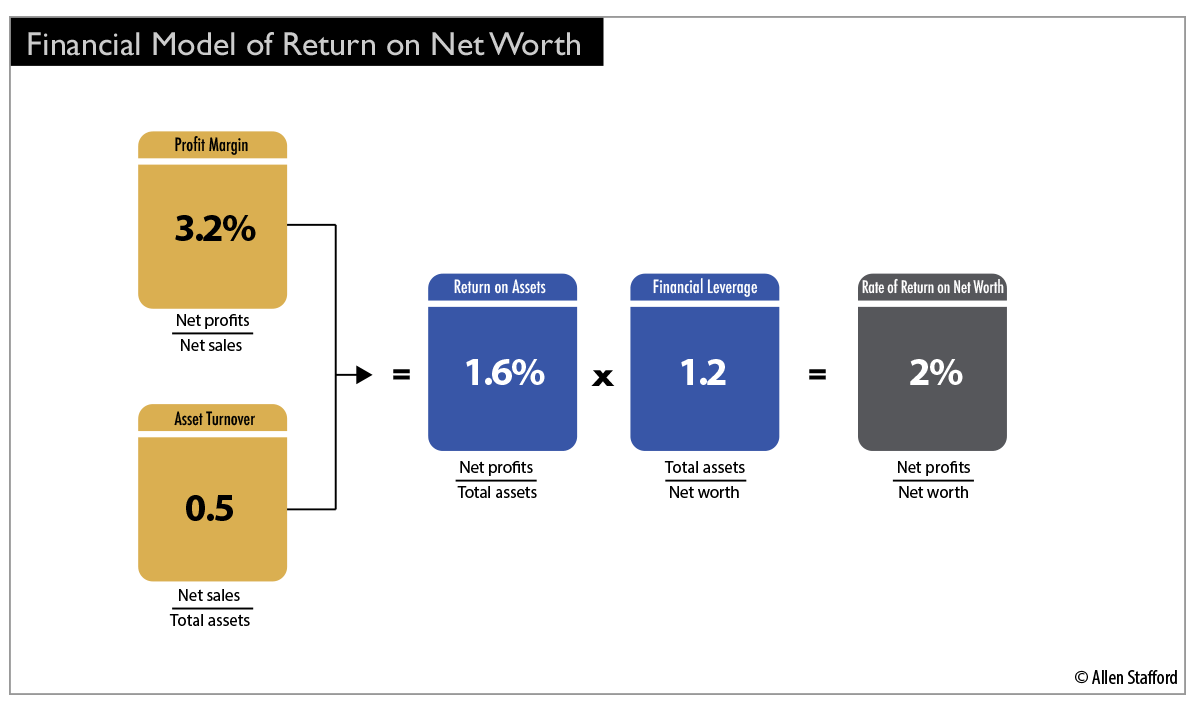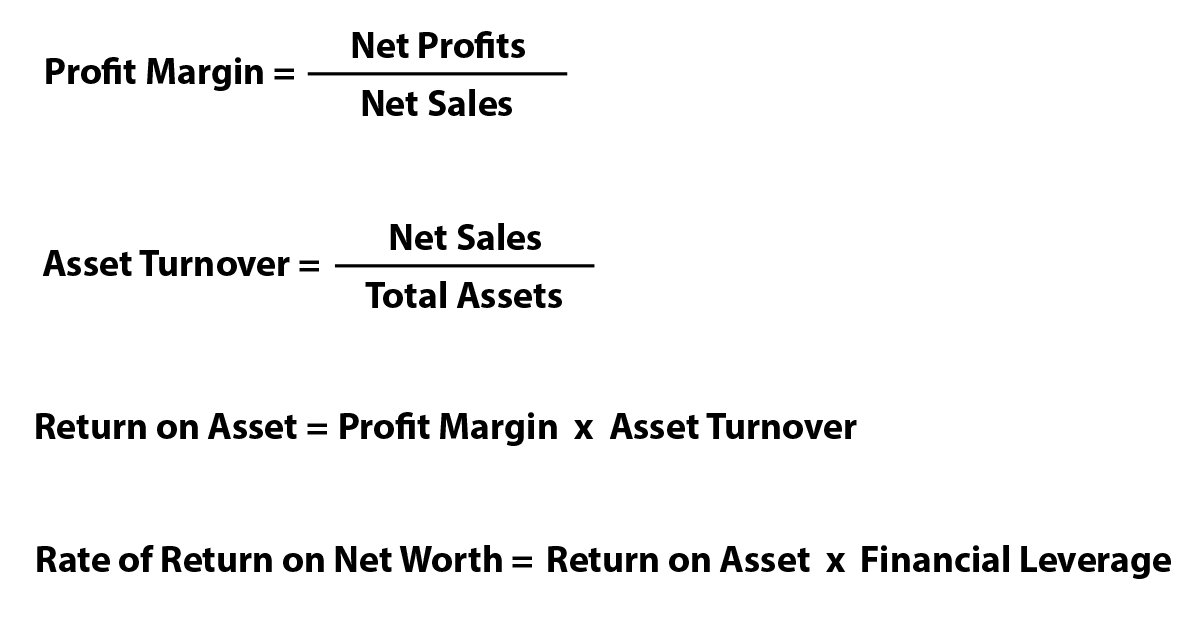# Financial Analysis Control Tool

Audio Version — 5:15

## Financial Analysis Control Tool Explained

The fourth tool for measuring the annual control plan is the financial analysis control tool. This analysis tool looks at the relationship between the expense-to-sales analysis (ratio) and the overall financial framework to analyze where and how the company is making or losing money concerning marketing activities. In other words, it’s an analysis tool used to determine the efficiency of how a firm is using its assets to generate revenue.

Companies efficient at generating revenue from their assets have a high asset turnover ratio. Whereas the opposite is true for firms that are not efficiently using their assets to generate sales, they have a low asset ratio.

Marketing managers and executives use the financial analysis tool to measure the return rate on their net worth.

The return on net worth is the product of two ratios: its return on assets and its financial leverage. The critical factors in determining the rate of return on net worth include:

• Profit margin (net profits / net sales)
• Asset turnover (net sales / total assets)
• Return on assets (net profits / total assets)
• Financial leverage (total assets / net worth)

There are two ways a company can improve the return on its net worth. They can:

1. Increase its ratio of net profits to assets.
2. Increase the ratio of assets to net worth.

Let’s take a look at an example of the financial analysis tool at work.

## Financial Analysis Example

I will use a utility company for the financial analysis example. Utility companies strive to have an asset turnover ratio between 0.25 and 0.5. The higher the asset ratio, the more efficient the firm is in generating revenue or sales from assets. As a side note, utility and manufacturing firms often have a more extensive asset base, which results in a lower asset turnover. On the other hand, retail businesses tend to have small asset bases with a higher sales volume that lends itself to a high asset turnover ratio.

Taking a look at the diagram below — the Financial Model of Return on Net Wort — we can see that the asset turnover for our utility company is 0.5, which is within a normal range for a utility company. However, its profit margin is low at 3.2%, whereas the average profit margin for a utility firm hovers around 9%.

### Financial Model of Return on Net Worth Formula Diagram## How to Improve Rate of Return on Net Worth

Before I discuss improving performance concerning the low-profit margin and average asset turnover in the utility company example, let’s address how the firm could improve its rate of return on net worth. I’ll examine the net worth first since the rate of return on net worth relates to the firm’s profit margin and asset turnover and the return on assets and financial leverage.

It’s important to note that the return on assets is the product of the profit margin and asset turnover ratio. The rate of return on net worth is the product of the return on assets and financial leverage. See the formula and calculation below for more information.

If our rate of return of net worth is 2% in our example, then for a utility company, it appears low. To improve its return on net worth, the utility company must do one of two things in this scenario. They must increase their ratio of net profits to assets or increase the proportion of assets to net worth. To achieve their goal, they should analyze their asset composition to determine if it can improve its asset management. Some assets may include:

• cash
• accounts receivable
• inventory
• plant and equipment

As discussed above, the return on assets is the product of the profit margin and asset turnover ratios. Because the asset turnover, in our example, appears to be in the normal range for utility companies, but the profit margin is low, the marketing executive can improve performance by increasing the:

1. Profit margin by increasing sales or cutting costs.
2. Asset turnover by increasing sales or reducing assets (inventory and receivables) held against a certain level of sales.

## Financial Analysis Calculations

There are several calculations for arriving at the Rate of Return on Net Worth in the diagram below. Use the Financial Model of Return on Net Worth above as a model for using the formulas below.## Summary

The financial analysis control tool is simple to use when calculating if a firm efficiently uses its assets to generate sales. A high asset ratio indicates that the company is financially efficient, and a low asset ratio indicates the opposite. The tool helps guide marketing executives who want to improve financial performance concerning sales for their companies. Two ways executives can improve performance. First, they can increase the profit margin by increasing sales through marketing programs or cutting costs to produce the product. Second, they can increase the asset turnover increasing sales volume or reducing assets such as inventory and receivables held against a certain level of sales.

Tags:
0 replies